# 二叉树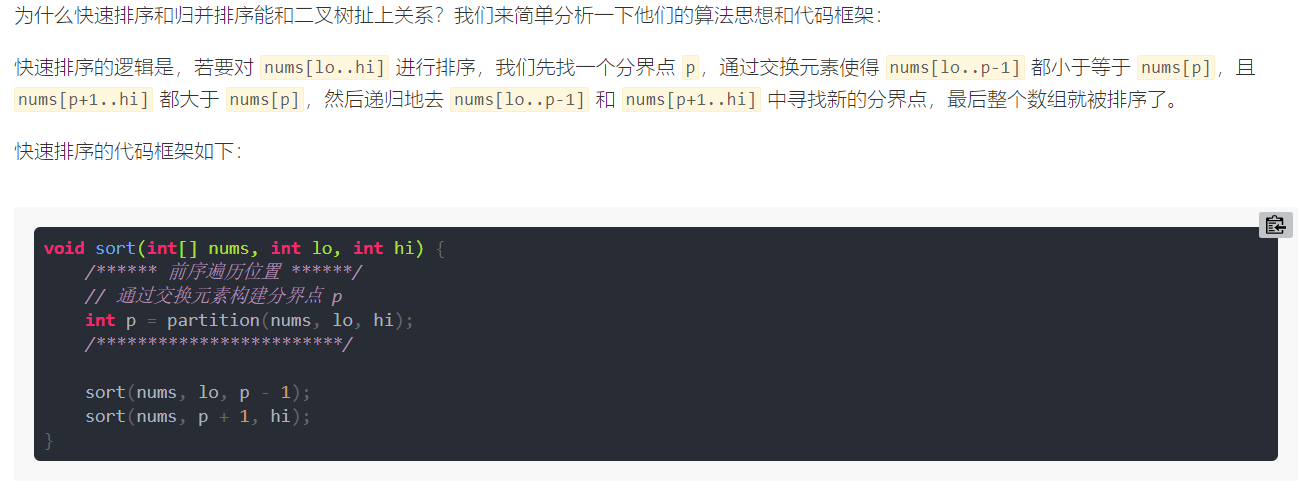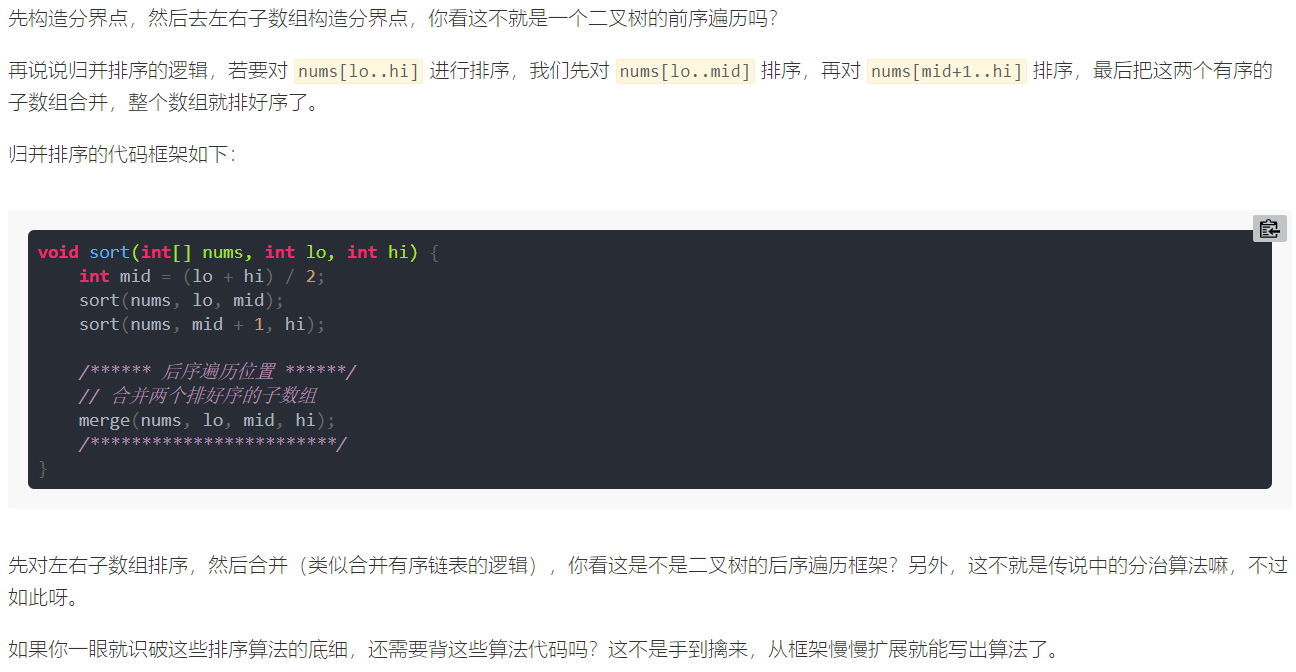## 226-翻转二叉树

`LeetCode`-226-简单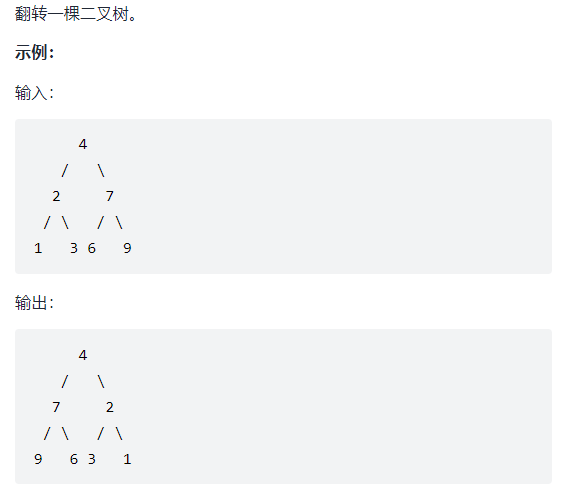``````public class invertTree_226 {
/**
* 翻转一颗二叉树
* */
public TreeNode invertTree(TreeNode root) {
// base case
if (root == null) {
return null;
}

// 前序遍历
TreeNode tmp = root.left;
root.left = root.right;
root.right = tmp;

// 让左右子节点继续翻转其子节点
invertTree(root.left);
invertTree(root.right);

return root;
}
}
``````

## 116-填充每个节点的下一个右侧节点指针

`LeetCode`-116-中等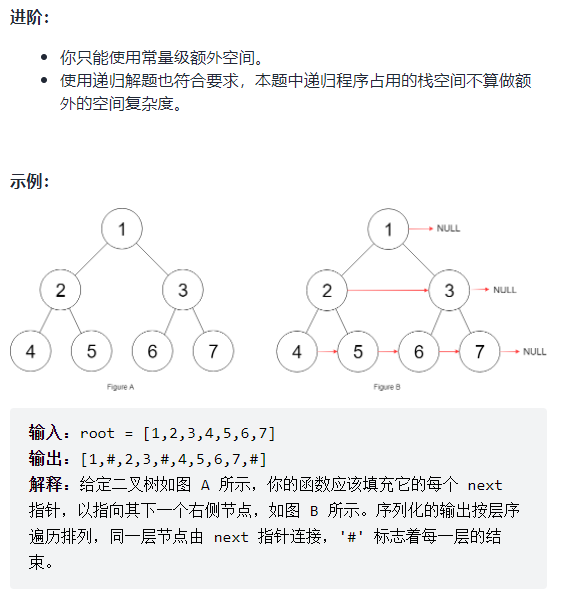``````public class connect_116 {

class Node {
public int val;
public Node left;
public Node right;
public Node next;

public Node() {}

public Node(int _val) {
val = _val;
}

public Node(int _val, Node _left, Node _right, Node _next) {
val = _val;
left = _left;
right = _right;
next = _next;
}
};

public Node connect(Node root) {
if (root == null) { return null; }
connectTwoNode(root.left, root.right);

return root;
}

void connectTwoNode(Node node1, Node node2) {
if (node1 == null || node2 == null) { return; }
// 前序遍历
node1.next = node2;
// 连接相同父节点的两个子节点
connectTwoNode(node1.left, node1.right);
connectTwoNode(node2.left, node2.right);
// 连接跨越父节点的两个子节点
connectTwoNode(node1.right, node2.left);
}
}
``````

## 114-二叉树展开为链表

`LeetCode`-114-中等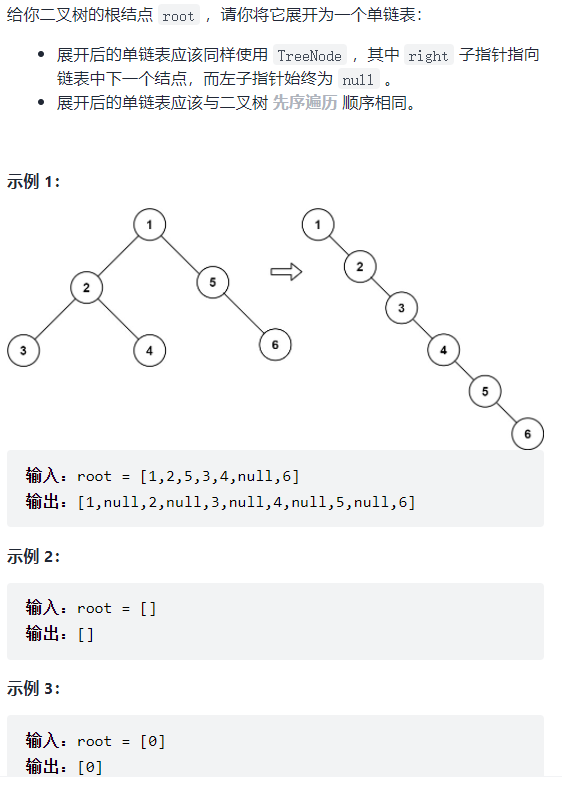`flatten` 函数输入一个节点 `root`，那么以 `root` 为根的二叉树就会被拉平为一条链表

1、将 `root` 的左子树和右子树拉平。

2、将 `root` 的右子树接到左子树下方，然后将整个左子树作为右子树。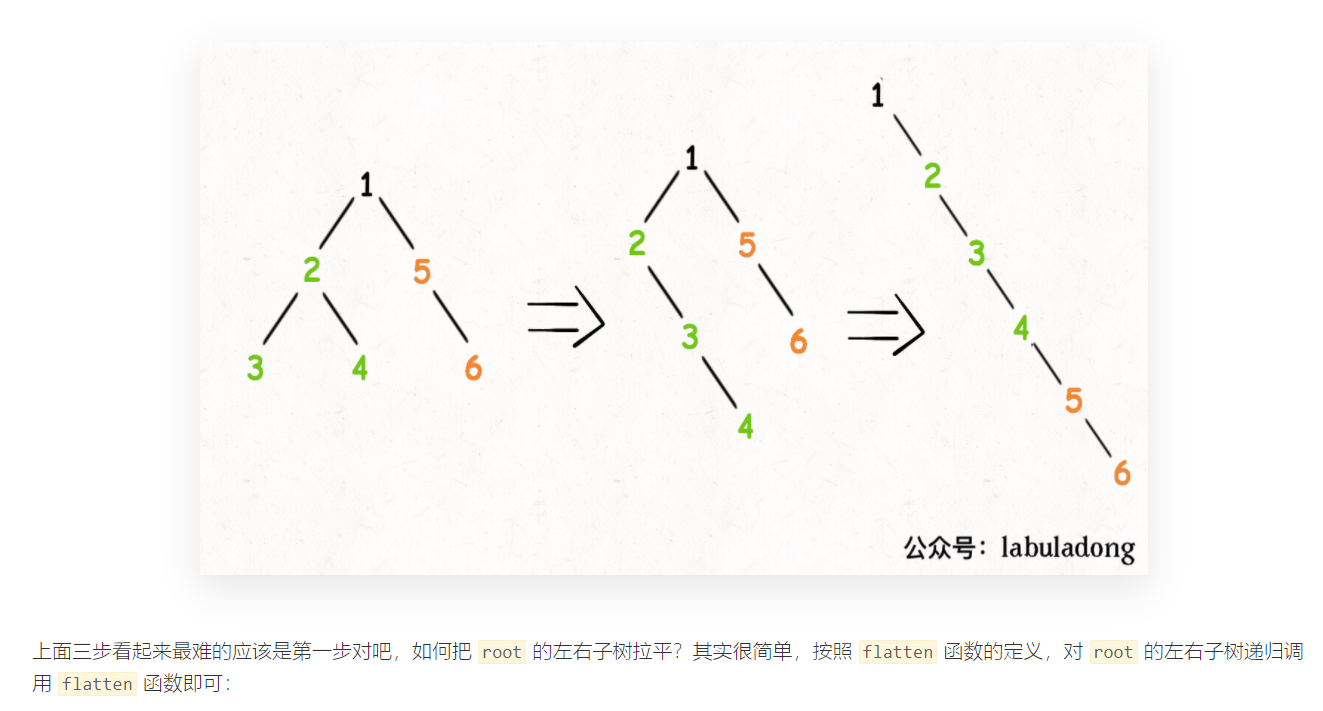``````public class flatten_114 {

public void flatten(TreeNode root) {
// base case
if (root == null) { return; }
// 将左右子树拉平
flatten(root.left);
flatten(root.right);
// 后续遍历
// 先保存左右子树
TreeNode left = root.left;
TreeNode right = root.right;
// 将左子树作为右子树
root.left = null;
root.right = left;
// 最后将右子树连接到左子树的末端
TreeNode p = root;
while (p != null) {
p = p.right;
}
p.right = right;
}
}
``````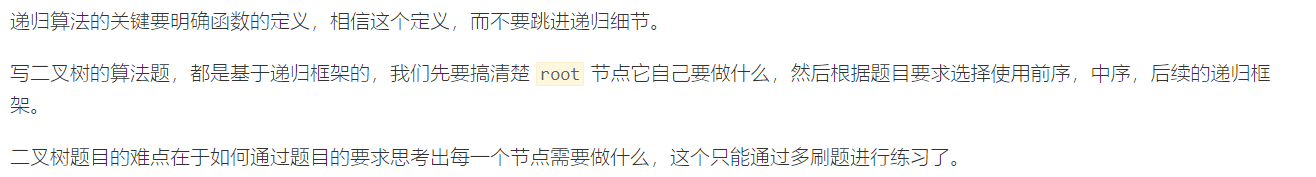## 654-构造最大二叉树

`LeetCode-654-中等`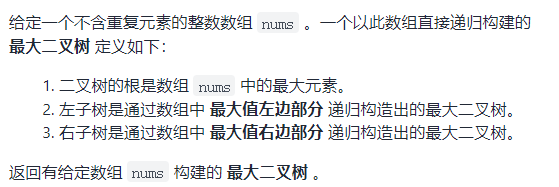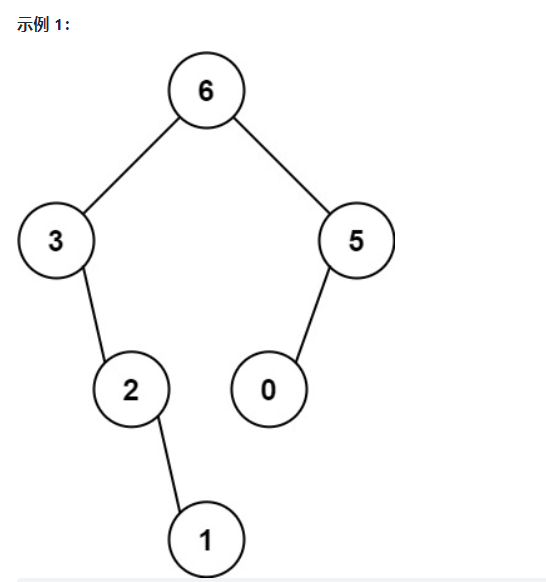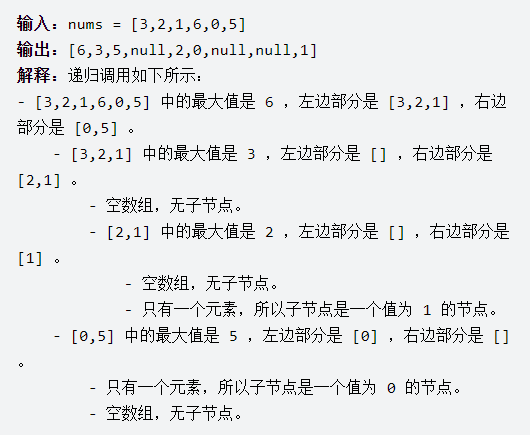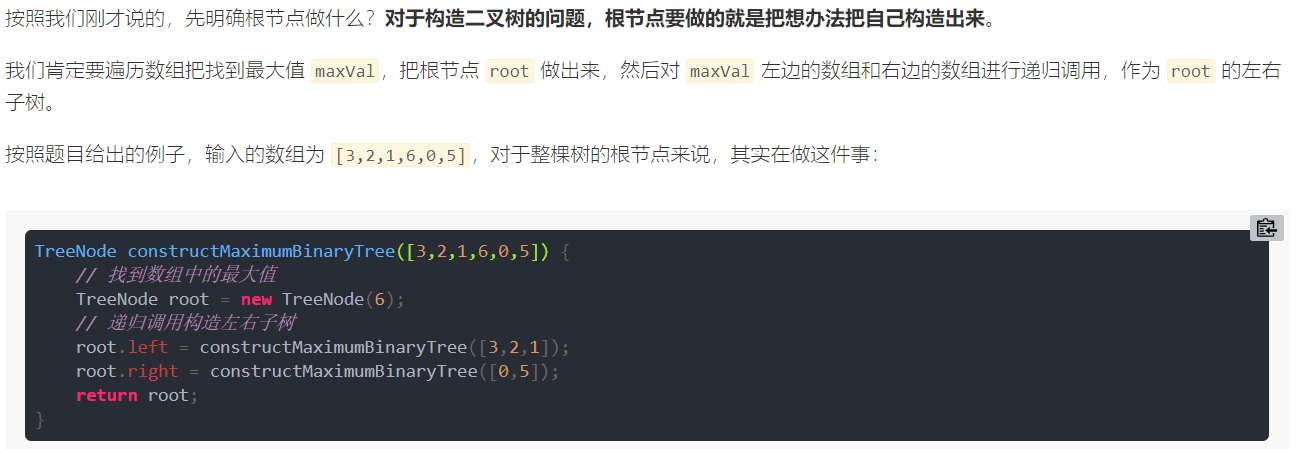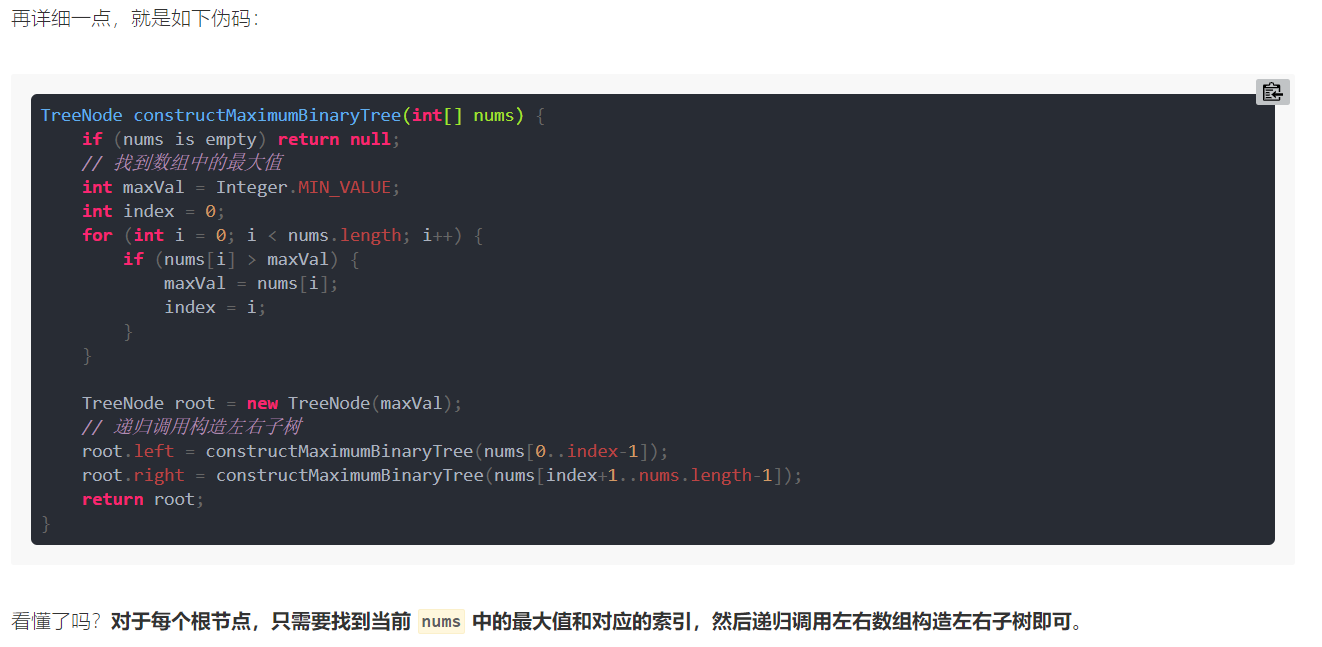``````public class constructMaximumBinaryTree_654 {

void traverse(TreeNode root) {
if (root == null) {
return;
}
System.out.println(root.val);
traverse(root.left);
traverse(root.right);
}

public static void main(String[] args) {
int[] nums = {3, 2, 1, 6, 0, 5};
constructMaximumBinaryTree_654 cmb = new constructMaximumBinaryTree_654();
cmb.traverse(cmb.constructMaximumBinaryTree(nums));
}

/**
* 最大二叉树
* */
public TreeNode constructMaximumBinaryTree(int[] nums) {
return build(nums, 0, nums.length-1);
}

TreeNode build(int[] nums, int lo, int hi) {
// base case
if (lo > hi) {
return null;
}

// 找到数组中的最大值和对应的索引
int index = -1, maxVal = Integer.MIN_VALUE;
for (int i = lo; i <= hi; i++) {
if (maxVal < nums[i]) {
maxVal = nums[i];
index = i;
}
}
// 前序遍历
TreeNode root = new TreeNode(maxVal);
// 递归调用构造左右子树
root.left = build(nums, lo, index-1);
root.right = build(nums, index+1, hi);

return root;
}
}
``````

## 105-从前序与中序遍历序列构造二叉树

`LeetCode-105-中等`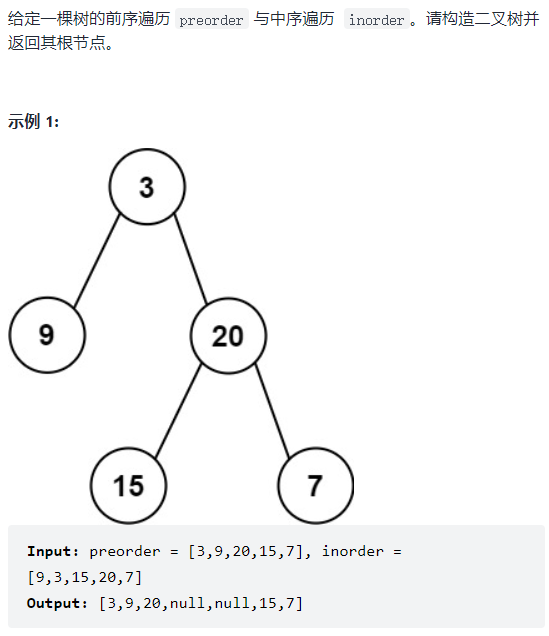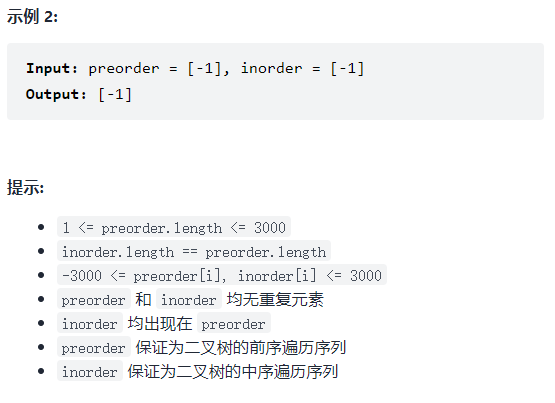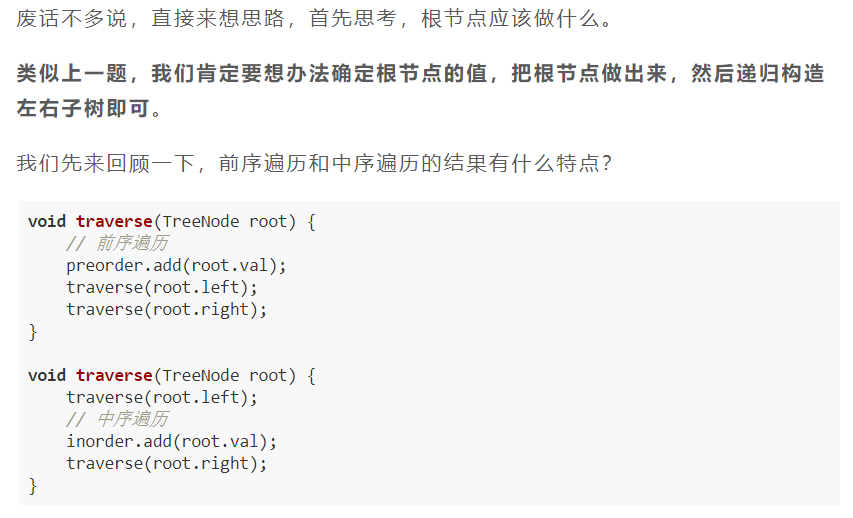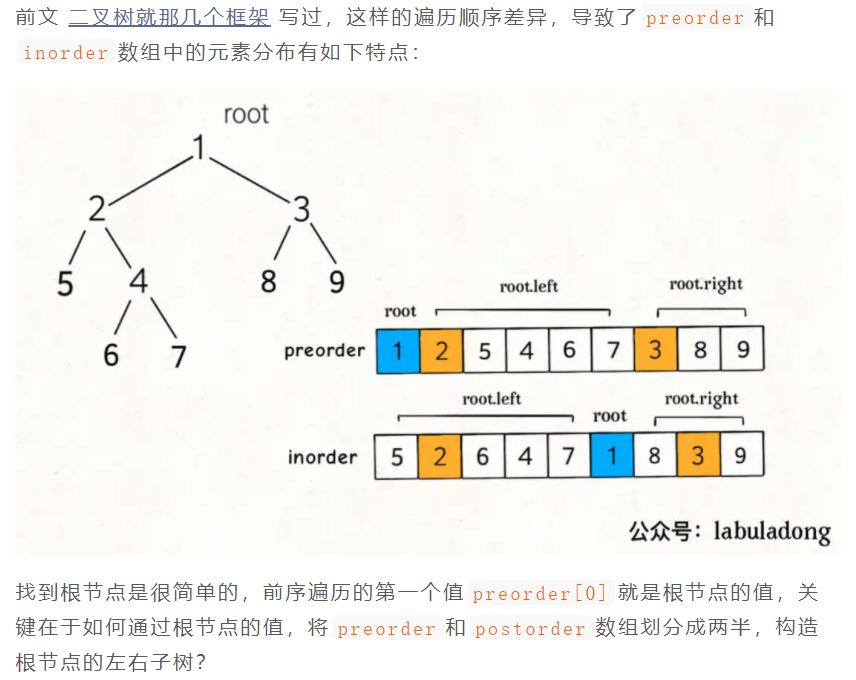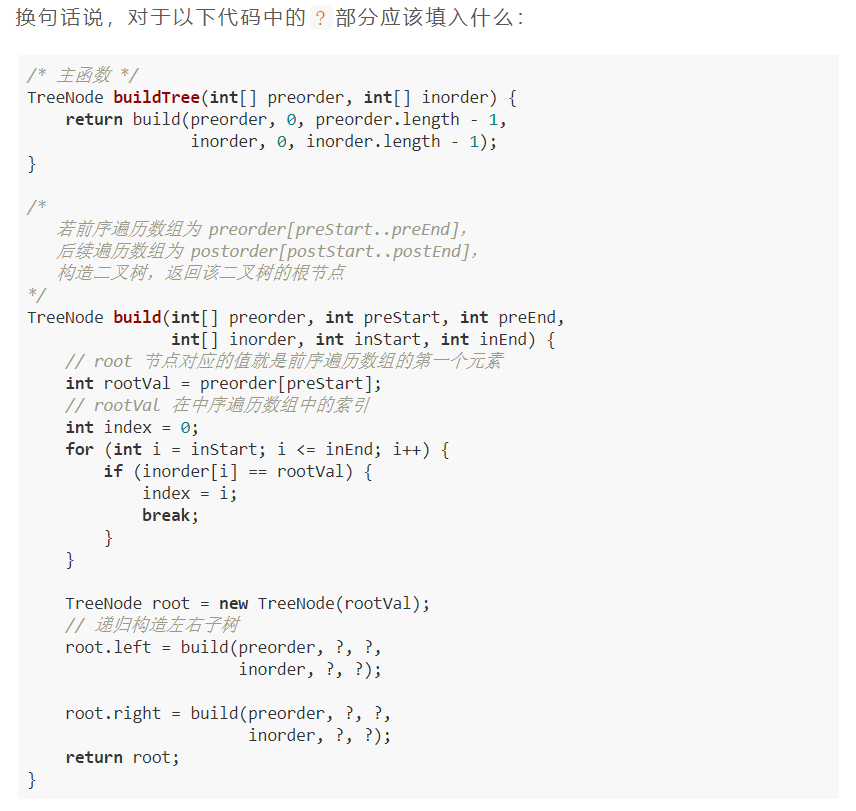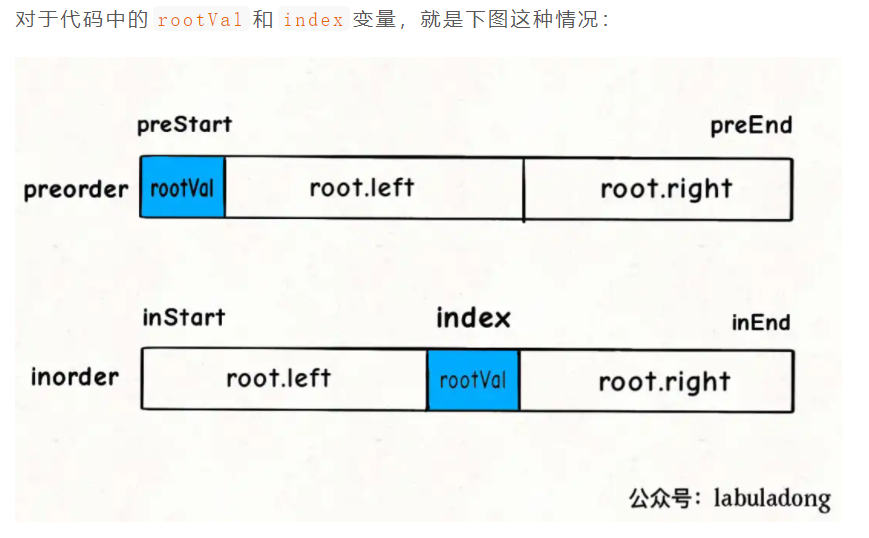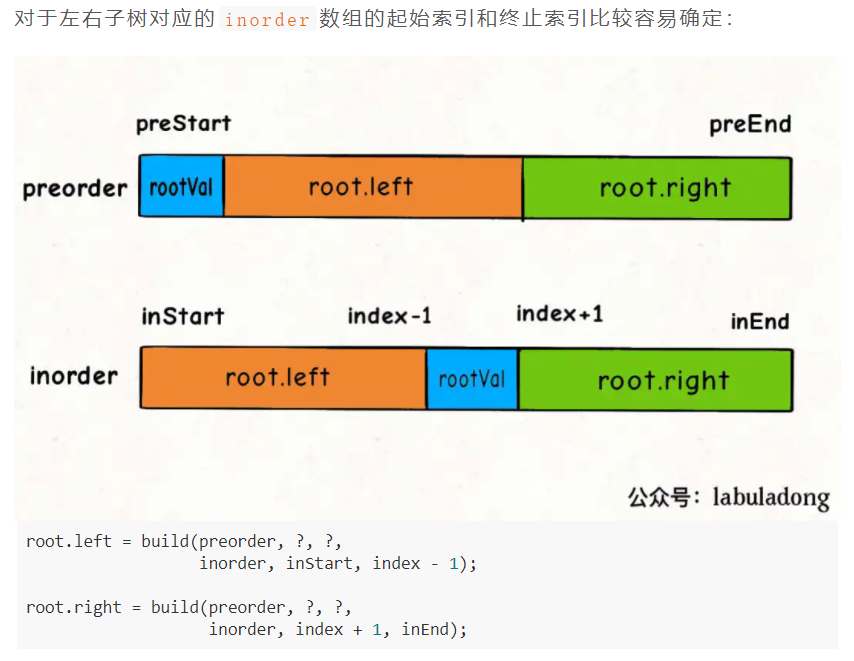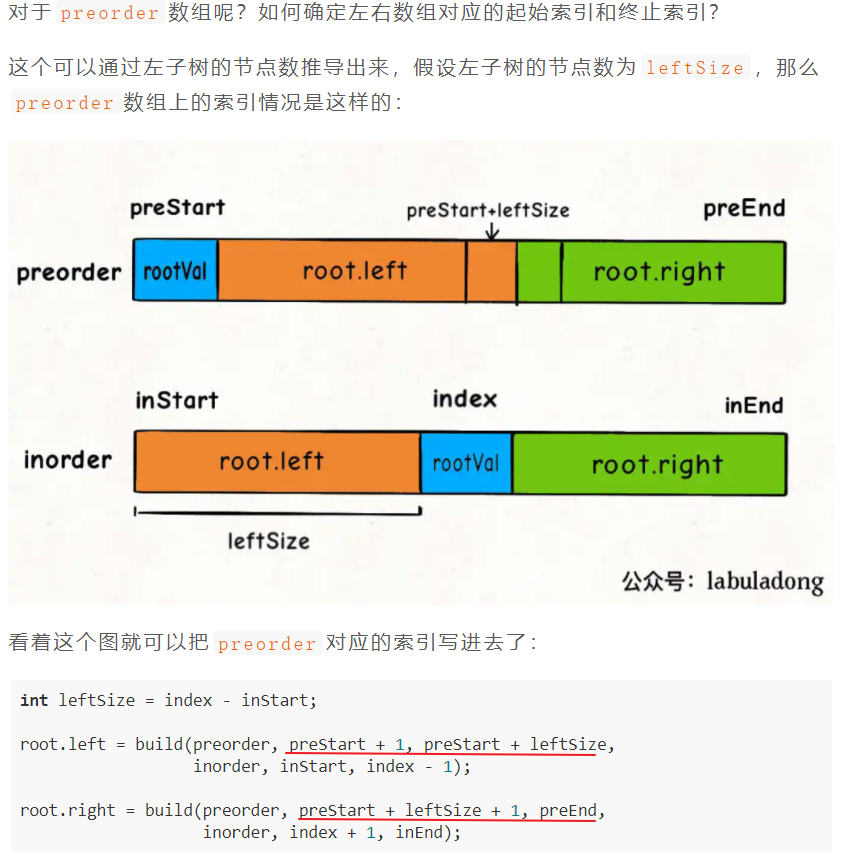``````TreeNode build(int[] preorder, int preStart, int preEnd,
int[] inorder, int inStart, int inEnd) {

if (preStart > preEnd) {
return null;
}

// root 节点对应的值就是前序遍历数组的第一个元素
int rootVal = preorder[preStart];
// rootVal 在中序遍历数组中的索引
int index = 0;
for (int i = inStart; i <= inEnd; i++) {
if (inorder[i] == rootVal) {
index = i;
break;
}
}

int leftSize = index - inStart;

// 先构造出当前根节点
TreeNode root = new TreeNode(rootVal);
// 递归构造左右子树
root.left = build(preorder, preStart + 1, preStart + leftSize,
inorder, inStart, index - 1);

root.right = build(preorder, preStart + leftSize + 1, preEnd,
inorder, index + 1, inEnd);
return root;
}
``````

``````public class buildTree_105 {
/**
* 给定一棵树的前序遍历 preorder 与中序遍历  inorder。
* 请构造二叉树并返回其根节点。
* 注意: 你可以假设树中没有重复的元素
* */
public TreeNode buildTree(int[] preorder, int[] inorder) {
return build(preorder, 0, preorder.length-1,
inorder, 0, inorder.length-1);
}

/**
* 递归构造
*/
private TreeNode build(int[] preorder, int preStart, int preEnd,
int[] inorder, int inStart, int inEnd) {
// base case
if (preStart > preEnd) {
return null;
}

// root 对应的值 及 在 中序遍历中的索引
int rootVal = preorder[preStart];
int index = preStart;
for (int i = inStart; i <= inEnd; i++) {
if (rootVal == inorder[i]) {
index = i;
break;
}
}

TreeNode root = new TreeNode(rootVal);
int len_left = index - inStart;
root.left = build(preorder, preStart+1, preStart+len_left,
inorder, inStart, index-1);
int len_right = inEnd - index;
root.right = build(preorder, preEnd-len_right+1, preEnd,
inorder, index+1, inEnd);

return root;
}
}
``````

## 106-从中序与后序遍历序列构造二叉树

`LeetCode-106-中等`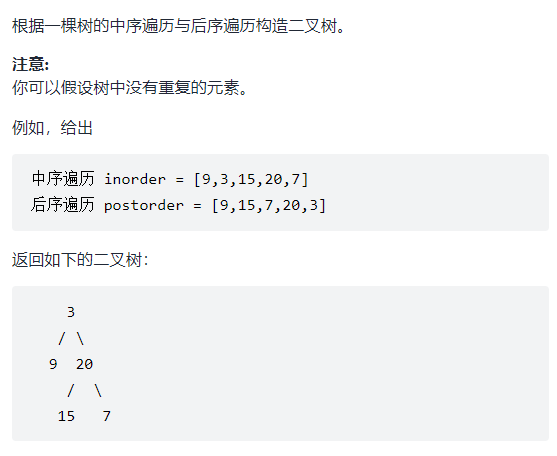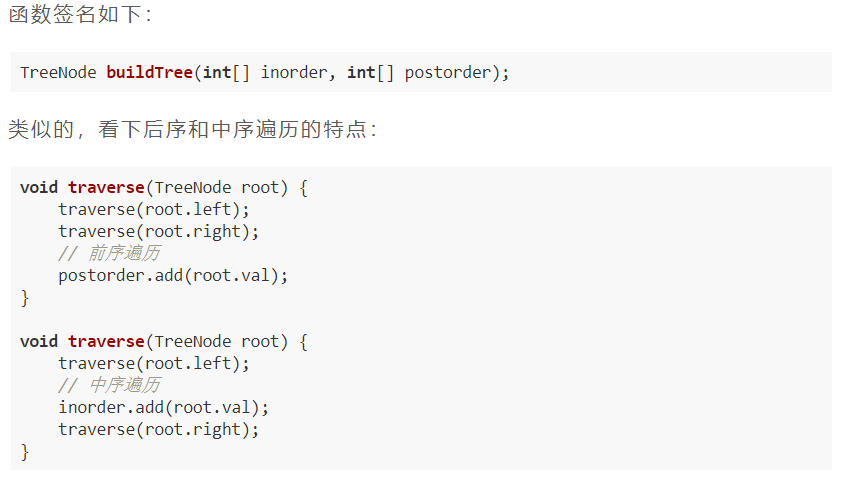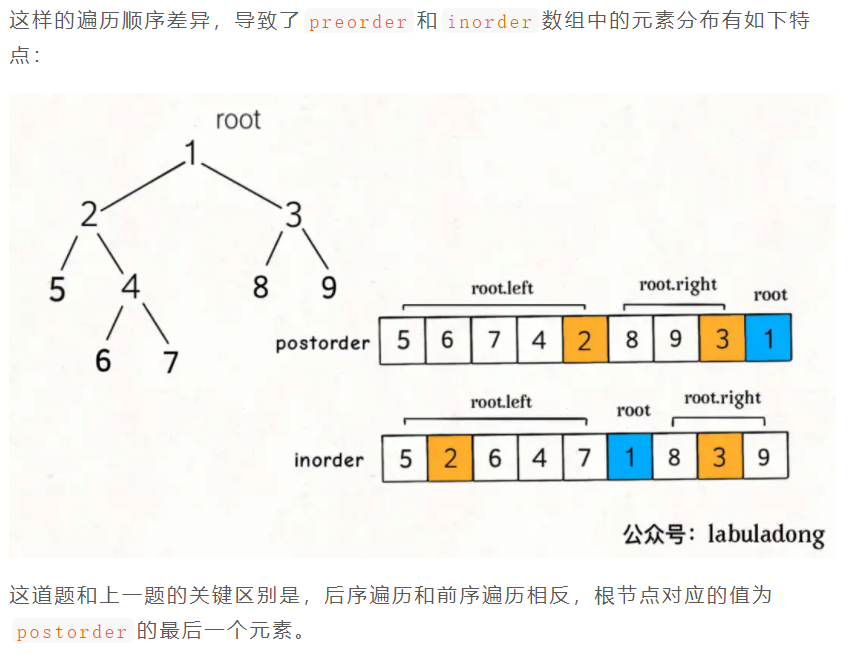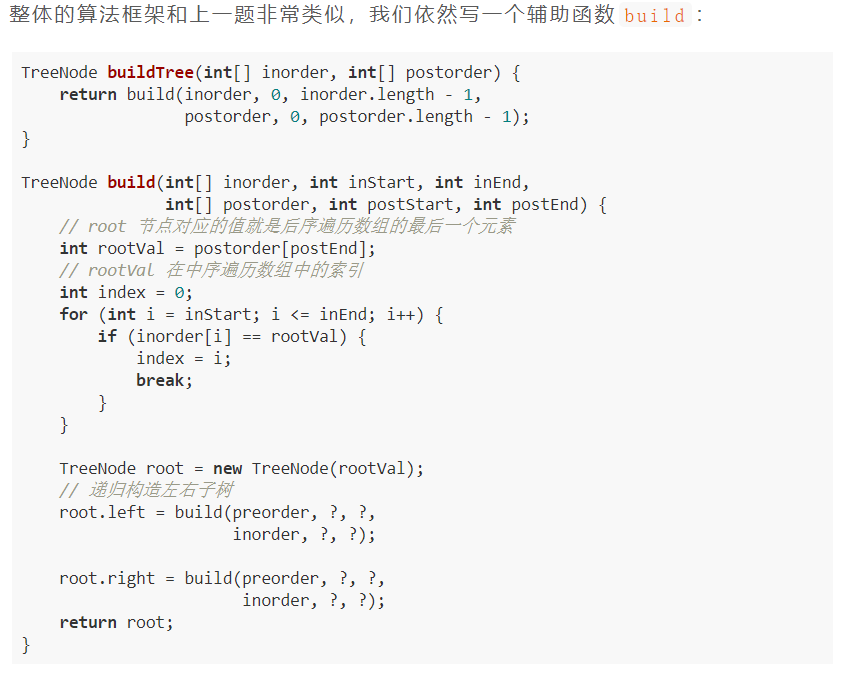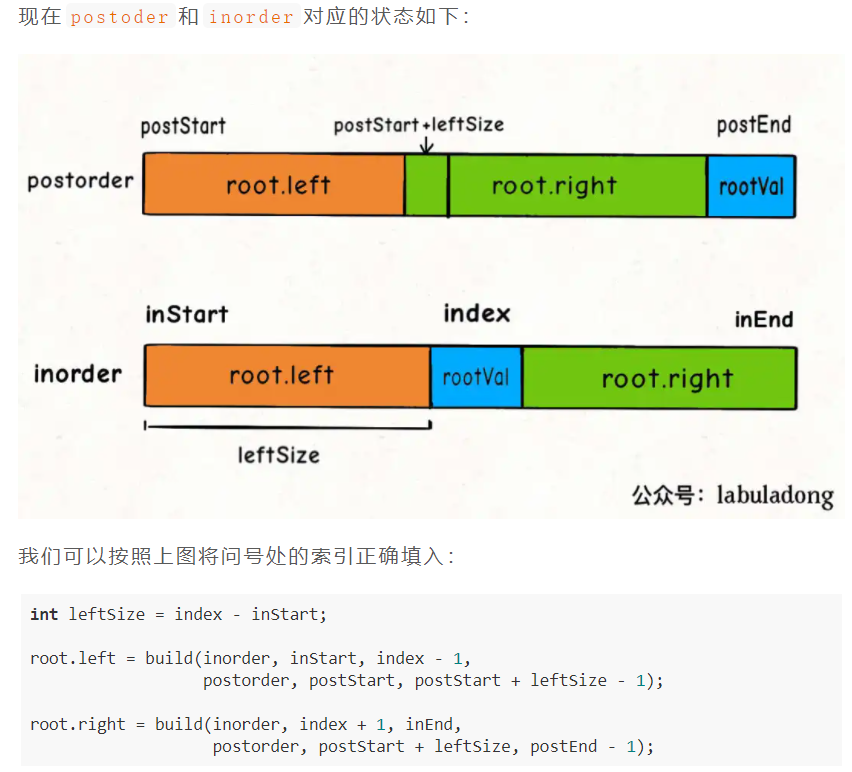``````TreeNode build(int[] inorder, int inStart, int inEnd,
int[] postorder, int postStart, int postEnd) {

if (inStart > inEnd) {
return null;
}
// root 节点对应的值就是后序遍历数组的最后一个元素
int rootVal = postorder[postEnd];
// rootVal 在中序遍历数组中的索引
int index = 0;
for (int i = inStart; i <= inEnd; i++) {
if (inorder[i] == rootVal) {
index = i;
break;
}
}
// 左子树的节点个数
int leftSize = index - inStart;
TreeNode root = new TreeNode(rootVal);
// 递归构造左右子树
root.left = build(inorder, inStart, index - 1,
postorder, postStart, postStart + leftSize - 1);

root.right = build(inorder, index + 1, inEnd,
postorder, postStart + leftSize, postEnd - 1);
return root;
}
``````

``````public class buildTree_106 {
public TreeNode buildTree(int[] inorder, int[] postorder) {
return build(inorder, 0, inorder.length-1,
postorder, 0, postorder.length-1);
}

private TreeNode build(int[] inorder, int inStart, int inEnd,
int[] postorder, int postStart, int postEnd) {
// base case
if (postStart > postEnd) {
return null;
}

// 找到root对应的值和索引
int rootVal = postorder[postEnd];
int index = postEnd;
for (int i = inStart; i <= inEnd; i++) {
if (inorder[i] == rootVal) {
index = i;
break;
}
}

TreeNode root = new TreeNode(rootVal);
int len_left = index - inStart;
root.left = build(inorder, inStart, index-1,
postorder, postStart, postStart+len_left-1);
int len_right = inEnd - index;
root.right = build(inorder, index+1, inEnd,
postorder, postEnd-len_right, postEnd-1);

return root;
}
}
``````

## 104-二叉树的最大深度

``````    3
/ \
9  20
/  \
15   7
``````

``````//leetcode submit region begin(Prohibit modification and deletion)
/**
* Definition for a binary tree node.
* public class TreeNode {
*     int val;
*     TreeNode left;
*     TreeNode right;
*     TreeNode() {}
*     TreeNode(int val) { this.val = val; }
*     TreeNode(int val, TreeNode left, TreeNode right) {
*         this.val = val;
*         this.left = left;
*         this.right = right;
*     }
* }
*/
class Solution {
public int maxDepth(TreeNode root) {

}
}
//leetcode submit region end(Prohibit modification and deletion)
``````

1、是否可以通过遍历一遍二叉树得到答案？如果可以，用一个 `traverse` 函数配合外部变量来实现。

2、是否可以定义一个递归函数，通过子问题（子树）的答案推导出原问题的答案？如果可以，写出这个递归函数的定义，并充分利用这个函数的返回值。

3、无论使用哪一种思维模式，你都要明白二叉树的每一个节点需要做什么，需要在什么时候（前中后序）做

``````class Solution {
public int maxDepth(TreeNode root) {
if (root == null) {
return 0;
}

int leftMax = maxDepth(root.left);
int rightMax = maxDepth(root.right);
return Math.max(leftMax, rightMax) + 1;
}
}
``````

``````class Solution {
int depth = 0;
int res = 0;

public int maxDepth(TreeNode root) {
traverse(root);
return res;
}

void traverse(TreeNode root) {
if (root == null) {
// 到达叶子结点, 更新最大深度
res = Math.max(res, depth);
return;
}

depth++;
traverse(root.left);
traverse(root.right);
depth--;
}
}
``````

## 543-二叉树的直径

``````          1
/ \
2   3
/ \
4   5
``````

``````//leetcode submit region begin(Prohibit modification and deletion)
/**
* Definition for a binary tree node.
* public class TreeNode {
*     int val;
*     TreeNode left;
*     TreeNode right;
*     TreeNode() {}
*     TreeNode(int val) { this.val = val; }
*     TreeNode(int val, TreeNode left, TreeNode right) {
*         this.val = val;
*         this.left = left;
*         this.right = right;
*     }
* }
*/
class Solution {
public int diameterOfBinaryTree(TreeNode root) {

}
}
//leetcode submit region end(Prohibit modification and deletion)
``````

``````class Solution {

int maxDiameter = 0;

public int diameterOfBinaryTree(TreeNode root) {
maxDepth(root);
return maxDiameter;
}

int maxDepth(TreeNode root) {
if (root == null) {
return 0;
}

int leftMax = maxDepth(root.left);
int rightMax = maxDepth(root.right);
maxDiameter = Math.max(maxDiameter, leftMax+rightMax);

return 1 + Math.max(leftMax, rightMax);
}
}
``````

# 二叉搜索树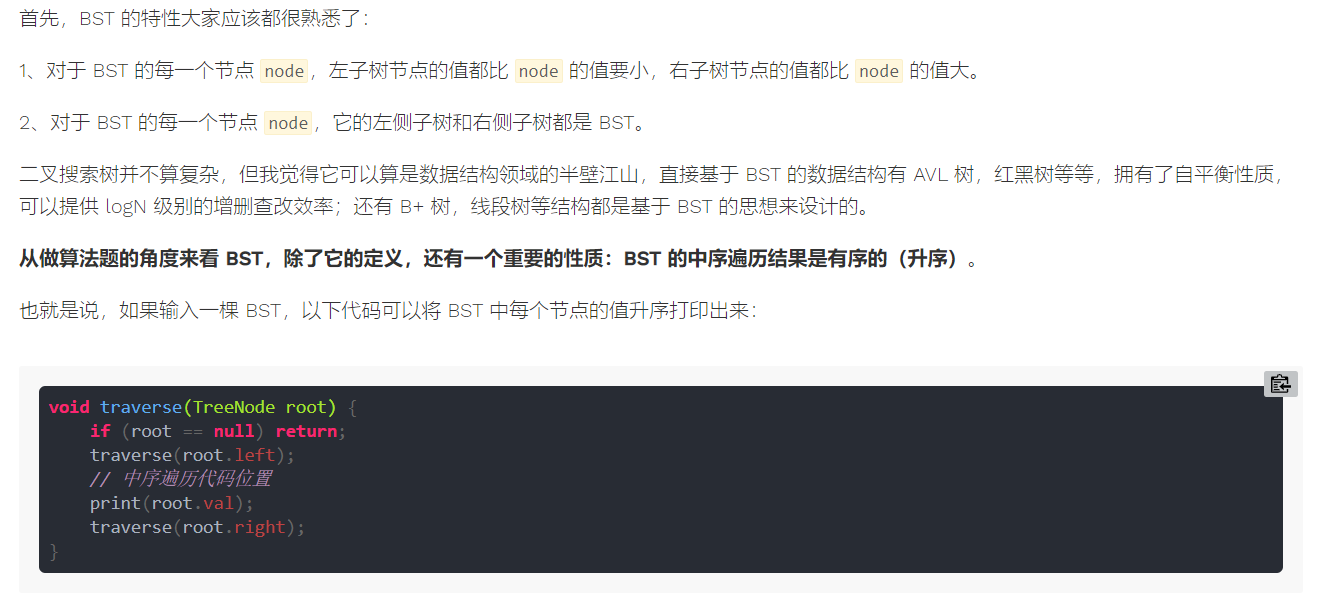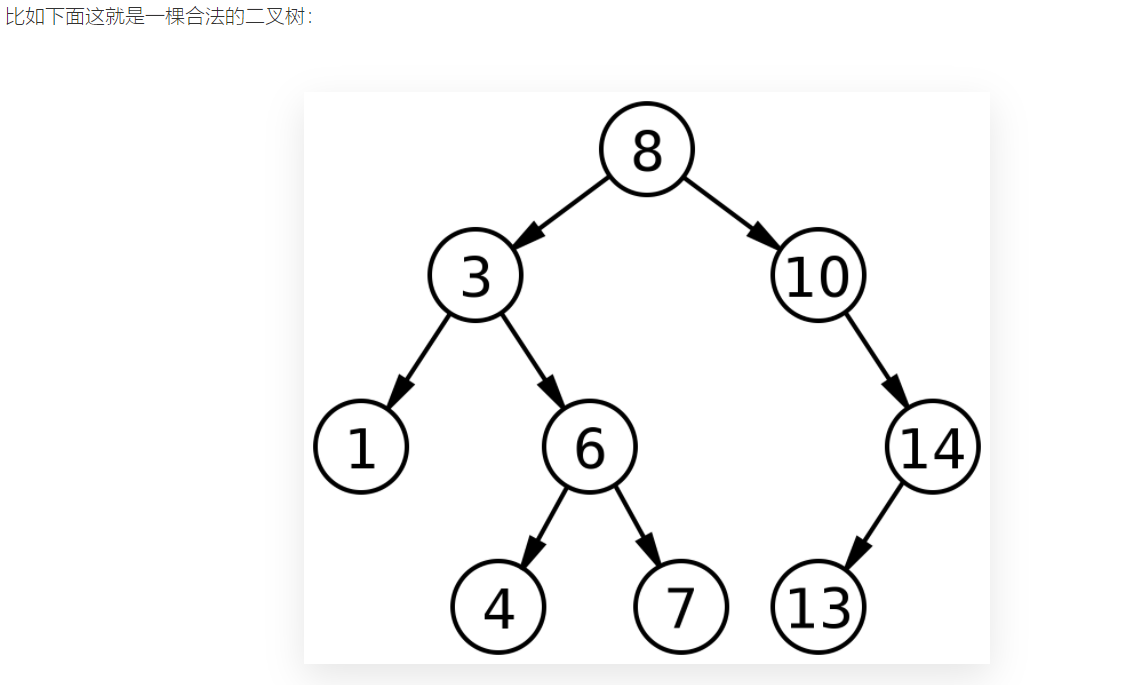``````void BST(TreeNode root, int target) {
if (root.val == target)
// 找到目标，做点什么
if (root.val < target)
BST(root.right, target);
if (root.val > target)
BST(root.left, target);
}
``````

## 230-BST第K小的元素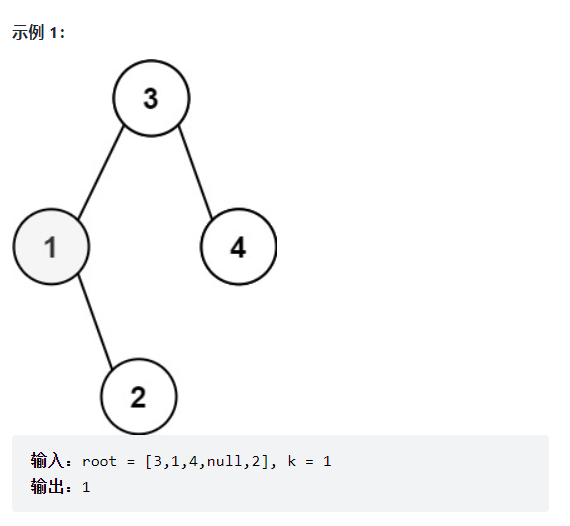``````/**
* Definition for a binary tree node.
* public class TreeNode {
*     int val;
*     TreeNode left;
*     TreeNode right;
*     TreeNode() {}
*     TreeNode(int val) { this.val = val; }
*     TreeNode(int val, TreeNode left, TreeNode right) {
*         this.val = val;
*         this.left = left;
*         this.right = right;
*     }
* }
*/
class Solution {
public int kthSmallest(TreeNode root, int k) {
traverse(root, k);
return res;
}

int res = 0;
int rank = 0;
void traverse(TreeNode root, int k) {
if (root == null) {
return;
}

traverse(root.left, k);

rank++;
if (k == rank) {
res = root.val;
return;
}

traverse(root.right, k);
}

}
``````

1、如果`m == k`，显然就是找到了第`k`个元素，返回当前节点就行了。

2、如果`k < m`，那说明排名第`k`的元素在左子树，所以可以去左子树搜索第`k`个元素。

3、如果`k > m`，那说明排名第`k`的元素在右子树，所以可以去右子树搜索第`k - m - 1`个元素。

``````class TreeNode {
int val;
// 以该节点为根的树的节点总数
int size;
TreeNode left;
TreeNode right;
}
``````

## 538/1038-把二叉搜索树转换为累加树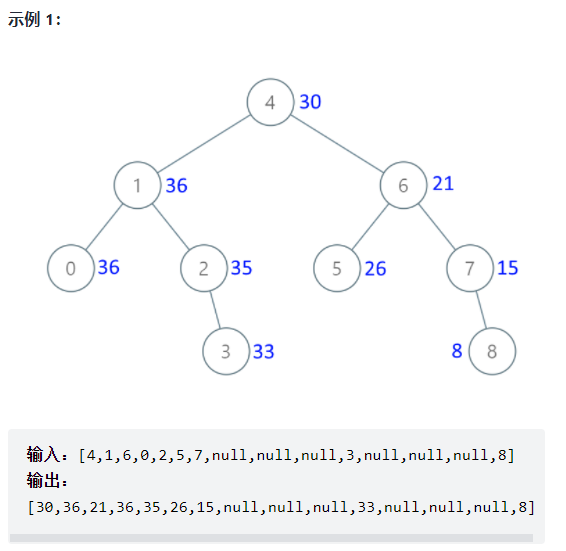BST 的每个节点左小右大，这似乎是一个有用的信息，既然累加和是计算大于等于当前值的所有元素之和，那么每个节点都去计算右子树的和，不就行了吗？

``````/**
* Definition for a binary tree node.
* public class TreeNode {
*     int val;
*     TreeNode left;
*     TreeNode right;
*     TreeNode() {}
*     TreeNode(int val) { this.val = val; }
*     TreeNode(int val, TreeNode left, TreeNode right) {
*         this.val = val;
*         this.left = left;
*         this.right = right;
*     }
* }
*/
class Solution {
public TreeNode convertBST(TreeNode root) {
traverse(root);

return root;
}

int sum = 0;

void traverse(TreeNode root) {
if (root == null) {
return;
}

traverse(root.right);

sum += root.val;
root.val = sum;

traverse(root.left);
}
}
``````

## 450-删除二叉搜索树中的节点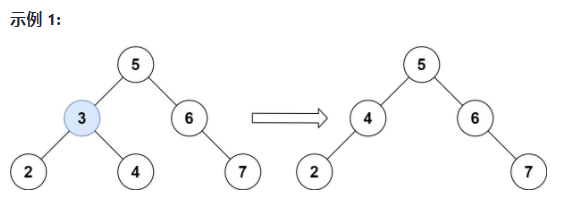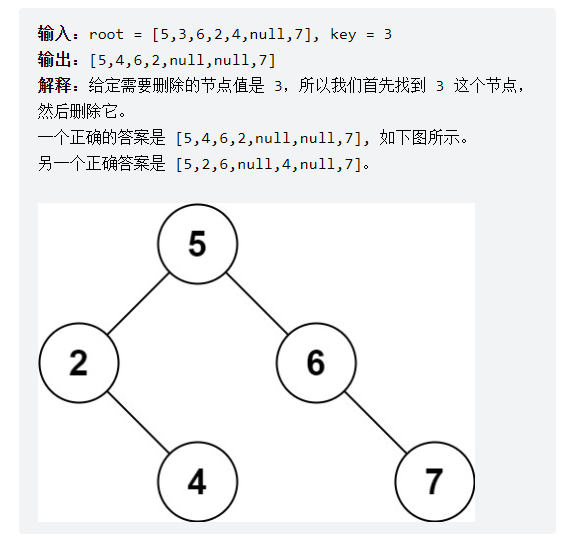``````TreeNode deleteNode(TreeNode root, int key) {
if (root.val == key) {
// 找到啦，进行删除
} else if (root.val > key) {
// 去左子树找
root.left = deleteNode(root.left, key);
} else if (root.val < key) {
// 去右子树找
root.right = deleteNode(root.right, key);
}
return root;
}
``````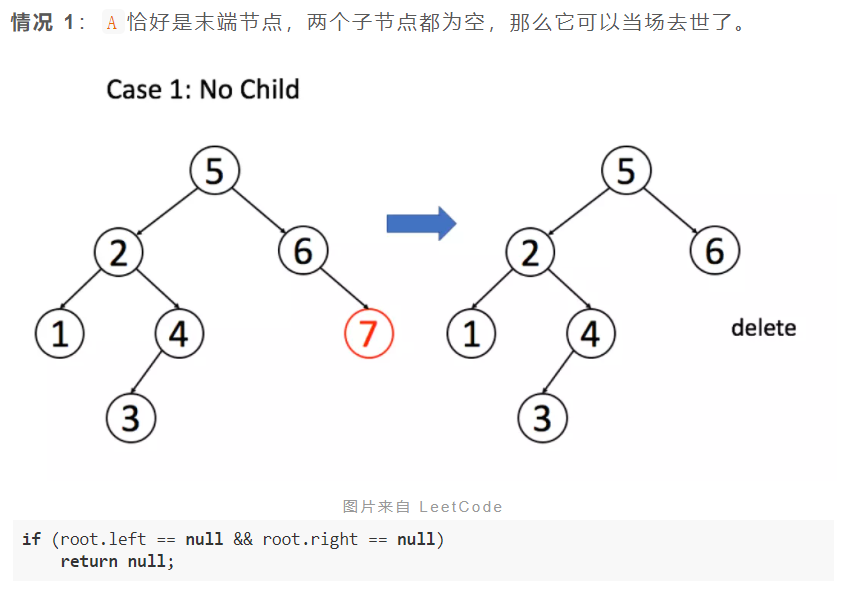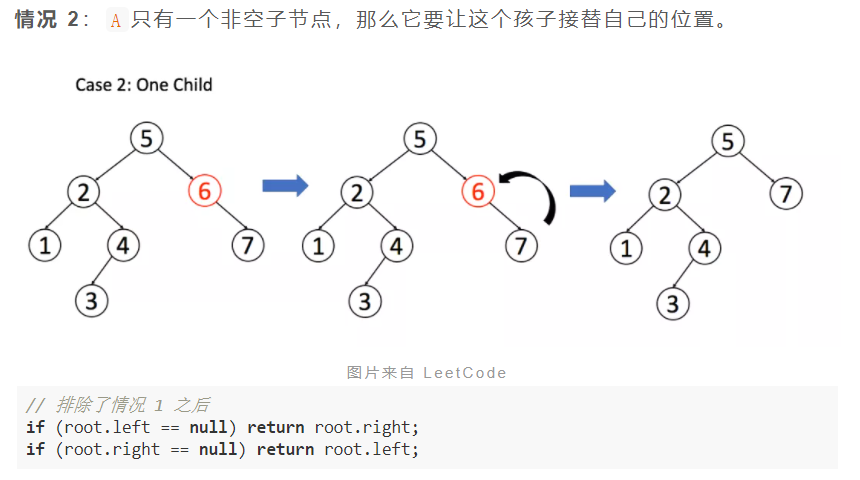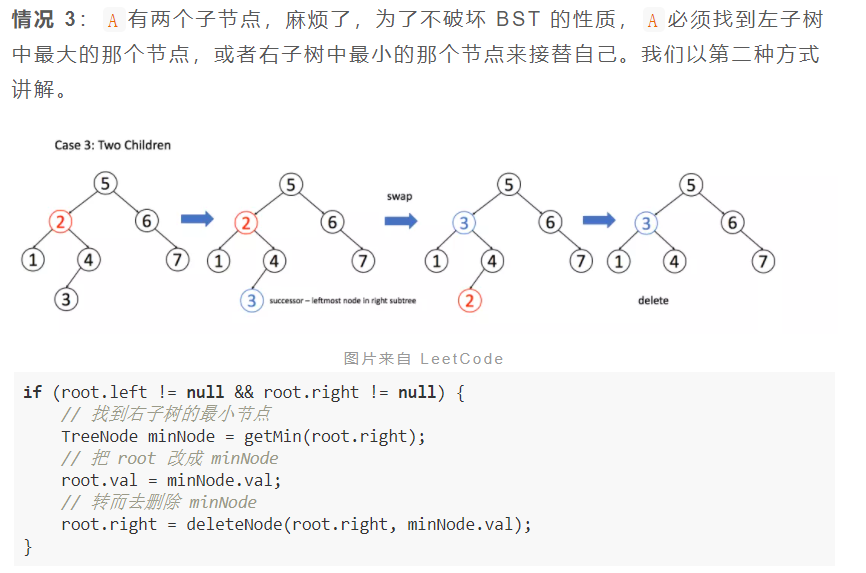``````/**
* Definition for a binary tree node.
* public class TreeNode {
*     int val;
*     TreeNode left;
*     TreeNode right;
*     TreeNode() {}
*     TreeNode(int val) { this.val = val; }
*     TreeNode(int val, TreeNode left, TreeNode right) {
*         this.val = val;
*         this.left = left;
*         this.right = right;
*     }
* }
*/
class Solution {
public TreeNode deleteNode(TreeNode root, int key) {
// base case
if (root == null) { return null; }

if (root.val == key) {
// 这两个 if 把情况 1 和 2 都正确处理了
if (root.left == null) { return root.right; }
if (root.right == null) { return root.left; }
// 情况3: 两个节点都有的情况 (获得右子树中最小的)
TreeNode minNode = getMin(root.right);
// 把 root 改成 minNode
root.val = minNode.val;
// 转而去删除 minNode
root.right = deleteNode(root.right, minNode.val);
} else if (root.val > key) {
// 去左子树找
root.left = deleteNode(root.left, key);
} else if (root.val < key) {
// 去右子树找
root.right = deleteNode(root.right, key);
}
return root;
}

/**
* 获得右子树中最小的
* */
TreeNode getMin(TreeNode node) {
// BST 最左边的就是最小的
while (node.left != null) {
node = node.left;
}
return node;
}
}
``````

## 701-二叉树中的插入操作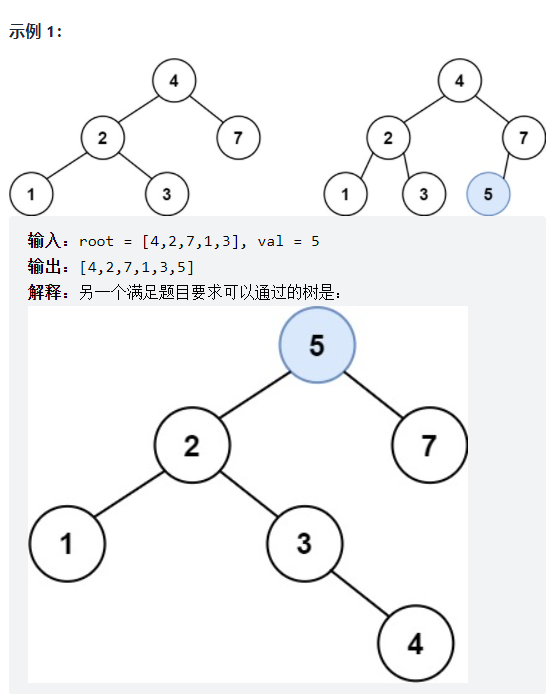``````class Solution {
TreeNode insertIntoBST(TreeNode root, int val) {
// 找到空位置插入新节点
if (root == null) return new TreeNode(val);

// if (root.val == val)
//     BST 中一般不会插入已存在元素
if (root.val < val)
root.right = insertIntoBST(root.right, val);
if (root.val > val)
root.left = insertIntoBST(root.left, val);
return root;
}
}
``````

## 700-二叉搜索树中的搜索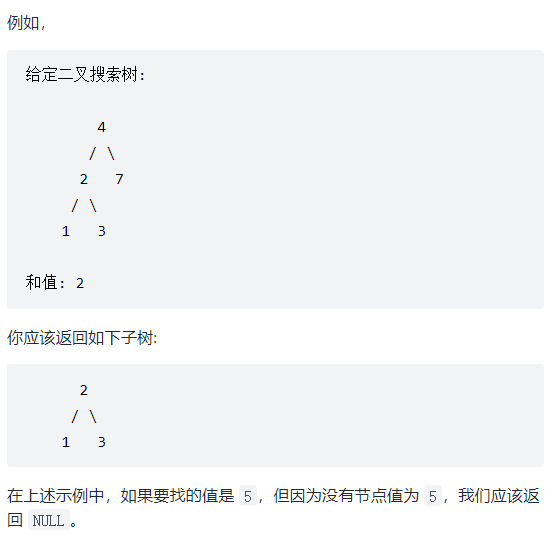``````class Solution {
public TreeNode searchBST(TreeNode root, int val) {
if (root == null) { return null; }

if (val == root.val) {
return root;
} else if (val < root.val) {
return searchBST(root.left, val);
} else if (val > root.val) {
return searchBST(root.right, val);
}

return null;
}
}
``````

``````class Solution {
TreeNode searchBST(TreeNode root, int target) {
if (root == null) {
return null;
}
// 去左子树搜索
if (root.val > target) {
return searchBST(root.left, target);
}
// 去右子树搜索
if (root.val < target) {
return searchBST(root.right, target);
}
return root;
}
}
``````

## 98-验证二叉搜索树

• 节点的左子树只包含 小于 当前节点的数。
• 节点的右子树只包含 大于 当前节点的数。
• 所有左子树和右子树自身必须也是二叉搜索树。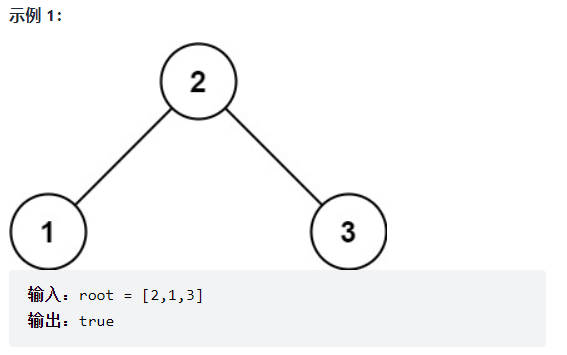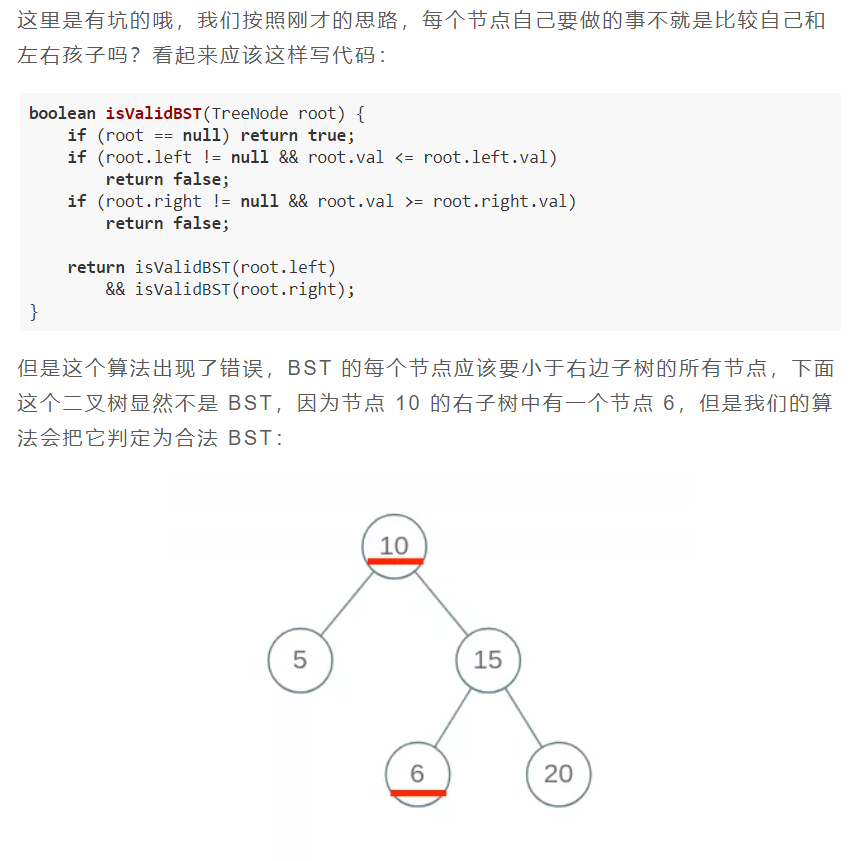**但是根据 BST 的定义，`root`的整个左子树都要小于`root.val`，整个右子树都要大于`root.val`**。

``````class Solution {
public boolean isValidBST(TreeNode root) {
return isValidBST(root, null, null);
}

private boolean isValidBST(TreeNode root, TreeNode min, TreeNode max) {
if (root == null) { return true; }

if (min != null && root.val <= min.val) { return false; }
if (max != null && root.val >= max.val) { return false; }

// 节点的左子树只包含 **小于** 当前节点的数。
// 节点的右子树只包含 **大于** 当前节点的数。
// 所有左子树和右子树自身必须也是二叉搜索树。
return isValidBST(root.left, min, root) && isValidBST(root.right, root, max);
}

}
``````

## 96-不同的二叉搜索树

https://leetcode-cn.com/problems/unique-binary-search-trees/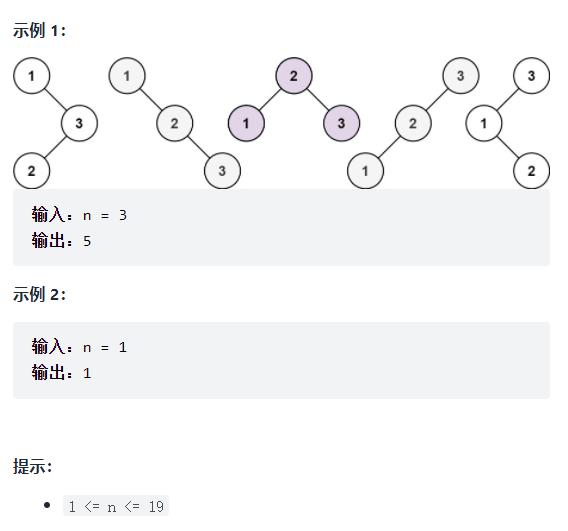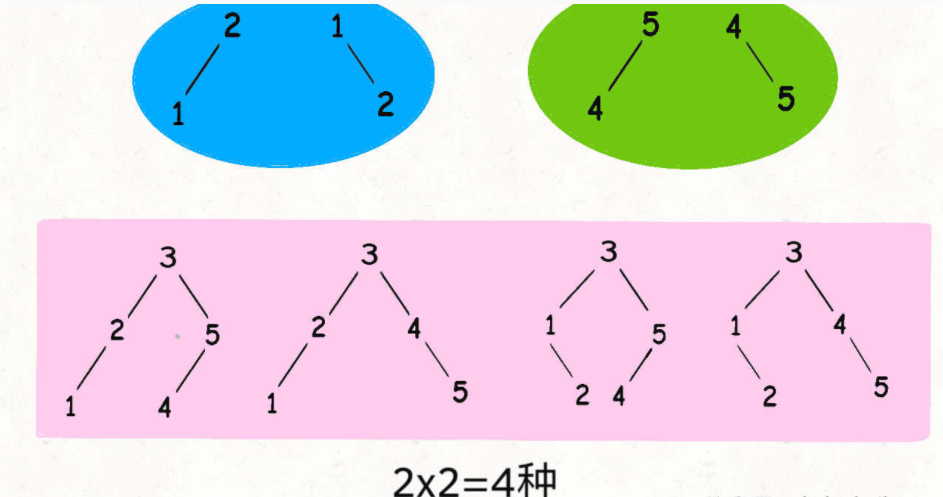``````class Solution {

/**
* 备忘录
* */
private int[][] memo;

public int numTrees(int n) {
// 备忘录的值初始化为 0
memo = new int[n+1][n+1];

// 计算闭区间 [1, n] 组成的 BST 个数
return count(1, n);
}

/**
* 计算闭区间 [lo, hi] 组成的 BST 个数
* */
int count(int lo, int hi) {
//base code
if (lo > hi) {
return 1;
}

// 查备忘录
if (memo[lo][hi] != 0) {
return memo[lo][hi];
}

int res = 0;
for (int i = lo; i <= hi; i++) {
// i 的值作为根节点 root
int left = count(lo, i-1);
int right = count(i+1, hi);
// 左右子树的组合数乘积是 BST 的总数
res += left * right;
}

// 将结果存入备忘录
memo[lo][hi] = res;

return res;
}
}
``````

## 95-不同的二叉搜索树 II

https://leetcode-cn.com/problems/unique-binary-search-trees-ii/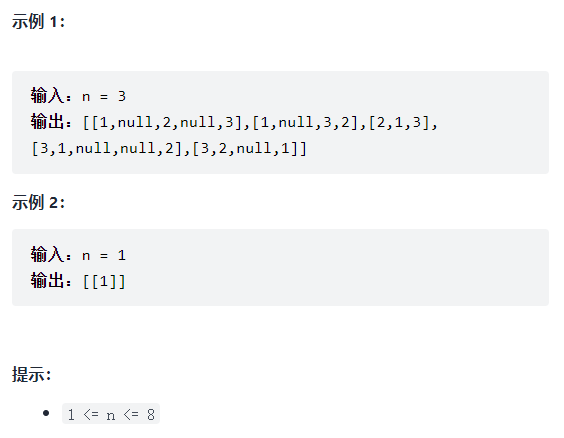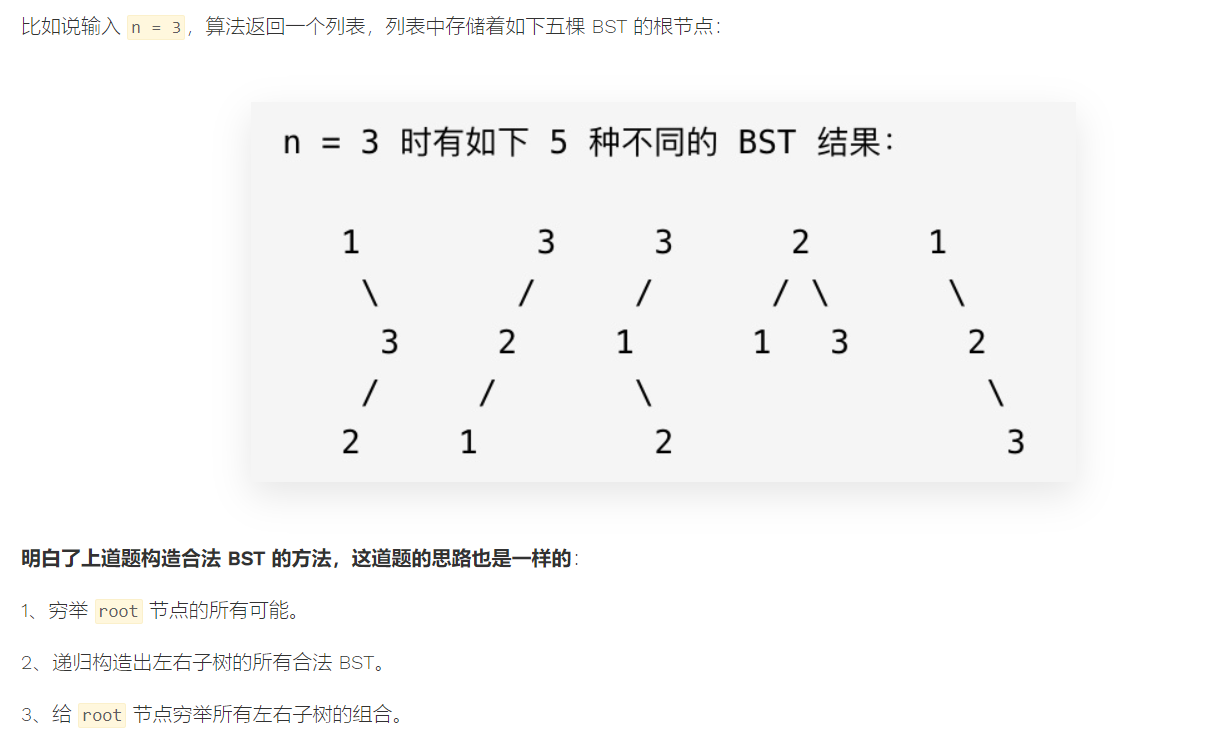``````/**
* Definition for a binary tree node.
* public class TreeNode {
*     int val;
*     TreeNode left;
*     TreeNode right;
*     TreeNode() {}
*     TreeNode(int val) { this.val = val; }
*     TreeNode(int val, TreeNode left, TreeNode right) {
*         this.val = val;
*         this.left = left;
*         this.right = right;
*     }
* }
*/
class Solution {
public List<TreeNode> generateTrees(int n) {
if (n == 0) {
}

// 构造闭区间 [1, n] 组成的 BST
return build(1, n);
}

/**
* 构造闭区间 [lo, hi] 组成的 BST
* */
private List<TreeNode> build(int lo, int hi) {

// base case
if (lo > hi) {
return res;
}

// 1 穷举 root 节点的所有可能
for (int i = lo; i <= hi; i++) {
// 2 递归构造出左右子树的所有合法BST
List<TreeNode> leftTree = build(lo, i-1);
List<TreeNode> rightTree = build(i+1, hi);
// 3 给 root 节点穷举所有左右子树的组合
for (TreeNode left : leftTree) {
for (TreeNode right : rightTree) {
// i 作为根节点 root 的值
TreeNode root = new TreeNode(i);
root.left = left;
root.right = right;
}
}
}

return res;
}

}
``````

## 1373-二叉搜索子树的最大键值和

https://leetcode-cn.com/problems/maximum-sum-bst-in-binary-tree/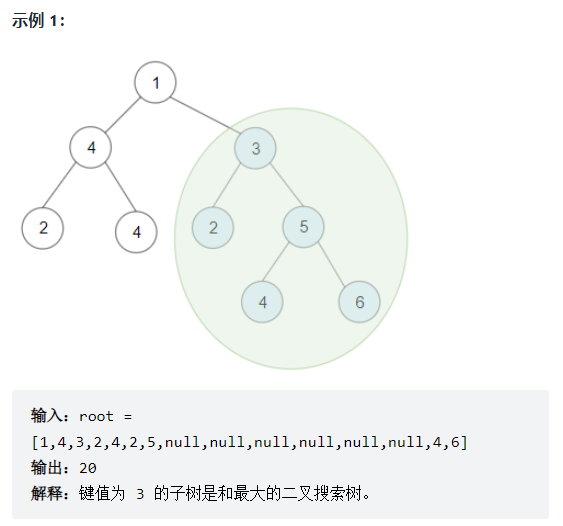• 每棵树有 `1``40000` 个节点。
• 每个节点的键值在 `[-4 * 10^4 , 4 * 10^4]` 之间。

``````void traverse(TreeNode root) {
traverse(root.left);
traverse(root.right);
/* 后序遍历代码的位置 */
/* 在这里处理当前节点 */
}
``````

1、我肯定得知道左右子树是不是合法的 BST，如果这俩儿子有一个不是 BST，以我为根的这棵树肯定不会是 BST，对吧。

2、如果左右子树都是合法的 BST，我得瞅瞅左右子树加上自己还是不是合法的 BST 了。因为按照 BST 的定义，当前节点的值应该大于左子树的最大值，小于右子树的最小值，否则就破坏了 BST 的性质。

3、因为题目要计算最大的节点之和，如果左右子树加上我自己还是一棵合法的 BST，也就是说以我为根的整棵树是一棵 BST，那我需要知道我们这棵 BST 的所有节点值之和是多少，方便和别的 BST 争个高下，对吧。

1、左右子树是否是 BST。

2、左子树的最大值和右子树的最小值。

3、左右子树的节点值之和。

``````// 全局变量，记录 BST 最大节点之和
int maxSum = 0;

/* 主函数 */
public int maxSumBST(TreeNode root) {
traverse(root);
return maxSum;
}

/* 遍历二叉树 */
void traverse(TreeNode root) {
if (root == null) {
return;
}

/******* 前序遍历位置 *******/
// 判断左右子树是不是 BST
if (!isBST(root.left) || !isBST(root.right)) {
goto next;
}
// 计算左子树的最大值和右子树的最小值
int leftMax = findMax(root.left);
int rightMin = findMin(root.right);
// 判断以 root 节点为根的树是不是 BST
if (root.val <= leftMax || root.val >= rightMin) {
goto next;
}
// 如果条件都符合，计算当前 BST 的节点之和
int leftSum = findSum(root.left);
int rightSum = findSum(root.right);
int rootSum = leftSum + rightSum + root.val;
// 计算 BST 节点的最大和
this.maxSum = Math.max(maxSum, rootSum);
/**************************/

// 递归左右子树
next:
traverse(root.left);
traverse(root.right);
}

/* 计算以 root 为根的二叉树的最大值 */
int findMax(TreeNode root) {}

/* 计算以 root 为根的二叉树的最小值 */
int findMin(TreeNode root) {}

/* 计算以 root 为根的二叉树的节点和 */
int findSum(TreeNode root) {}

/* 判断以 root 为根的二叉树是否是 BST */
boolean isBST(TreeNode root) {}
``````

`traverse(root)` 返回一个大小为 4 的 `int` 数组，我们暂且称它为 `res`，其中：

• `res` 记录以 `root` 为根的二叉树是否是 BST，若为 1 则说明是 BST，若为 0 则说明不是 BST；
• `res` 记录以 `root` 为根的二叉树所有节点中的最小值；
• `res` 记录以 `root` 为根的二叉树所有节点中的最大值；
• `res` 记录以 `root` 为根的二叉树所有节点值之和。

``````class Solution {
// 全局变量，记录 BST 最大节点之和
int maxSum = 0;

/* 主函数 */
public int maxSumBST(TreeNode root) {
traverse(root);
return maxSum;
}

// 函数返回 res = new int[] {isBST, min, max, sum};
int[] traverse(TreeNode root) {
// base case
if (root == null) {
return new int[]{1, Integer.MAX_VALUE, Integer.MIN_VALUE, 0};
}

// 递归计算左右子树
int[] left = traverse(root.left);
int[] right = traverse(root.right);

/******* 后序遍历位置 *******/
int[] res = new int;
// 这个 if 在判断以 root 为根的二叉树是不是 BST
if (left == 1 && right == 1
&& root.val > left && root.val < right) {
// 以 root 为根的二叉树是 BST
res = 1;
// 计算以 root 为根的这棵 BST 的最小值
res = Math.min(left, root.val);
// 计算以 root 为根的这棵 BST 的最大值
res = Math.max(right, root.val);
// 计算以 root 为根的这棵 BST 所有节点之和
res = left + right + root.val;
// 更新全局变量
maxSum = Math.max(maxSum, res);
} else {
// 以 root 为根的二叉树不是 BST
res = 0;
// 其他的值都没必要计算了，因为用不到
}

return res;
}
}
``````

×